### Home > CALC > Chapter 11 > Lesson 11.2.4 > Problem11-83

11-83.
1. Examine the integrals below. Consider the multiple tools available for integrating and use the best strategy. After evaluating each integral, write a short description of your method. Homework Help ✎

1.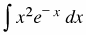2.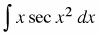3.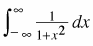4.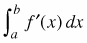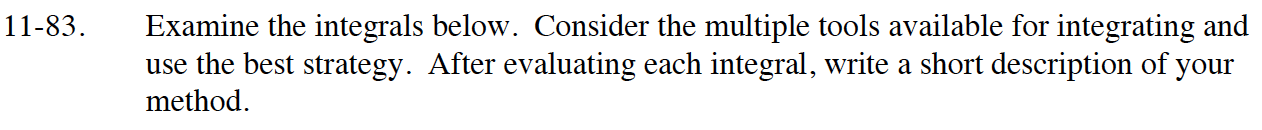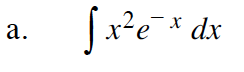Use integration by parts.
Let f = x2 and dg = ex.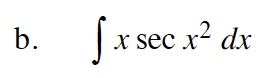Use substitution.
Let u = x2.

$\int\sec(x)dx=\ln|\sec(x)+\tan(x)|$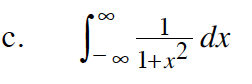You should recognize the integrand as the derivative of an inverse trigonometric function.

This is an improper integral, so a limit will need to be used to evaluate it.
The symmetry of the graph can also be used to evaluate the integral.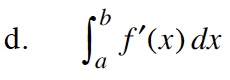Review the first Fundamental Theorem of Calculus.